## Thursday, March 31, 2011

### 462

462 = 2 x 3 x 7 x 11.

Assuming the Generalized Riemann Hypothesis, 462 is the largest number that is not of the form ab + bc + ca, for positive integers a, b, and c.

462462 + 462 + 1 is a prime number.

4622 = 213,444 = (21 - 3 + 444)2.

462 is 3322 in base 5; 462 is 2050 in base 6 and 250 in base 14; 462 is 110 in base 21.Mathematician Zu Chongzhi introduced the Daming calendar in China in the year 462.

## Wednesday, March 30, 2011

### 363

363 = 3 x 112.

363 is a divisor of 4010 - 1.

363 and the 363rd triangular number (66,066) are both palindromic.

3634 = 17,363,069,361 and 6304 = 173,630,693,610,000.

363 is 11223 in base 4, 2423 in base 5, and 123 in base 18; 363 is 300 in base 11; 363 is 553 in base 8 and 443 in base 9.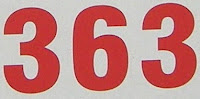On April 20 in the year 363, the planet Venus occulted the planet Jupiter.

Source: Numeropedia

## Tuesday, March 29, 2011

### 287

287 = 7 x 41.

287 is a number that cannot be written as a sum of three squares.

287 is the sum of consecutive primes in three different ways: 287 = 89 + 97 + 101 = 47 + 53 + 59 + 61 + 67 = 17 + 19 + 23 + 29 + 31 + 37 + 41 + 43 + 47.

287 is the sum of the first three NSW primes: 287 = 7 + 41 + 239.287 Nephthys is a large asteroid discovered in 1889.

## Monday, March 28, 2011

### 934

934 = 2 x 467.

934 has a fifth root that starts 3.25252225 . . .

934 is a number of the form n2 + n + 4, for n = 30.

934 is the number of positions that the 3 x 3 x 3 Rubik's cube can be in after exactly five moves (under the full group of order 48 of the cube). This is the number of positions that can be reached in five moves, but which cannot be reached in fewer than five moves.

934 is the sixteenth Fibonacci number minus the sixteenth prime number: 934 = 987 - 53.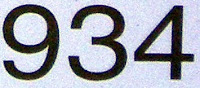The Porsche 934, introduced in 1976, was a racing version of the Porsche 911 Turbo.

## Friday, March 25, 2011

### 6119

6119 = 29 x 211.

6119 is a number that cannot be written as a sum of three squares.

6119 is a divisor of 6314 - 1.

6119 is a strobogrammatic number. It appears the same whether viewed normally or upside down.

6119 is 143434 in base 5.Lionel model trains included the D.L. & W. Work Caboose No. 6119 from 1955 to 1966.

## Thursday, March 24, 2011

### 628

628 = 22 x 157.

628 has a representation as sum of two squares: 628 = 122 + 222.

628 is 444 in base 12.

628 is the sum of the squares of four consecutive primes: 628 = 72 + 112 + 132 + 172.The Indian astronomer and mathematician Brahmagupta wrote the mathematics book The Opening of the Universe in the year 628.

## Wednesday, March 23, 2011

### 532

532 = 22 x 7 x 19.

532 has a unique representation as a sum of three squares: 532 = 82 + 122 + 182.

532 is 444 in base 11.

532 is a divisor of 116 - 1.

532 is the smallest even number differing by a composite number from both of its prime neighbors.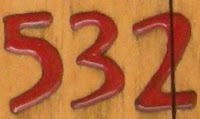532 was the first year in which the Anno Domini calendar was used for numbering the years.

## Tuesday, March 22, 2011

### 850

850 = 2 x 52 x 17.

850 has three representations as a sum of two squares: 850 = 32 + 292 = 112 + 272 = 152 + 252.

850 is the number of trees on 14 vertices with graph diameter 7.

850 is 2323 in base 7 and 1144 in base 9.850 is the maximum Fair Isaac credit score.

## Monday, March 21, 2011

### 419

419 is a prime number.

419 is also a Sophie Germain prime because 2 x 419 + 1 = 839 is also prime. It is the 22nd Sophie Germain prime, and 22 = 2 x 11 is the product of two Sophie Germain primes.

419 and 421 form a twin prime pair.

419 is a divisor of 1311 - 1.

419 is the smallest number that when divided by 2, 3, 4, 5, 6, and 7 leaves remainders of 1, 2, 3, 4, 5, and 6, respectively. Also, 419 is 1 less than the least common multiple of the first seven integers.

419, 41, and 19 are all primes. Their reversals, 914, 14, and 91, are all semiprimes.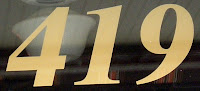Dutch naturalist and microscopy pioneer Anton van Leeuwenhoek (1632-1723) ground a total of 419 lenses during his life.

## Friday, March 18, 2011

### 601

601 is a prime number.

599 and 601 form a twin prime pair.

601 has a representation as a sum of two squares: 601 = 52 + 242.

601 is the hypotenuse of a primitive Pythagorean triple: 6012 = 2402 + 5512.

601 is the lesser member p of the least sexy prime pairs (p, p + 6) such that all numbers in between have the same number of prime divisors (counted with multiplicity).The Minivac 601 Digital Computer Kit was a product created by Claude Shannon and sold by Scientific Development Corporation as early as 1961.

## Thursday, March 17, 2011

### 500

500 = 22 x 53.

500 has two representations as a sum of two squares: 500 = 42 + 222 = 102 + 202.

There are exactly 500 prime numbers between 20,000 and 25,000.

166,500,333 = 1663 + 5003 + 3333.

500 is 4000 in base 5. It is 13310 in base 3 and 1313 in base 7.A ream contains 500 sheets of paper.

Source: Numeropedia

## Wednesday, March 16, 2011

### 433

433 is a prime number.

433 has a representation as a sum of two squares: 433 = 122 + 172.

431 and 433 form a twin prime pair.

433 is the hypotenuse of a primitive Pythagorean triple: 4332 = 1452 + 4082.

The last 433 digits of 433433 form a prime number.

4334 = 35152125121 and 035 + 152 + 125 + 121 = 433.433 Eros was the first Near-Earth asteroid to be discovered.

## Tuesday, March 15, 2011

### 195

195 = 3 x 5 x 13.

195 is 11000011 in base 2 (binary), 3003 in base 4, and 303 in base 8.

195 is the third integer that is the sum of the squares of three consecutive primes: 195 = 112 + 72 + 52.

195 is in the middle of the prime quadruplet 191, 193, 197, and 199.IC 195 is one member of a pair of galaxies in the constellation Aries.

## Monday, March 14, 2011

### 909

909 = 32 x 101.

909 has a representation as a sum of two squares: 909 = 32 + 302.

909 is a value of n that has no digits in common with 2n, 3n, 4n, 5n, 6n, 7n, 8n, or 9n.

9092 = 826,281 = (826 + 2 + 81)2.The Roland TR-909 was a drum machine produced in 1983.

## Friday, March 11, 2011

### 60

60 = 22 x 3 x 5.

60 is the smallest number with six representations as a sum of two primes: 60 = 7 + 53 = 13 + 47 = 17 + 43 = 19 + 41 = 23 + 27 = 29 + 31.

60 is a number that cannot be written as a sum of three squares.

60 is 66 ion base 9.

60 is the least common multiple of the first five positive integers.There are 60 seconds in one minute and 60 minutes in one hour.

Source: Phillips, R. 2004. Numbers: Facts, Figures and Fiction. Badsey Publications.

## Thursday, March 10, 2011

### 998

998 = 2 x 499.

998 is the smallest number with the property that its first 55 multiples contain the digit 9.

9983 = 994,011,992 = (994 + 0 + 1 + 1 + 9 - 9 + 2)3.

998 is 4342 in base 6 and 828 in base 11.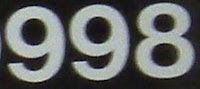The Ducati 998 motorcycle was produced from 2002 to 2004.

## Wednesday, March 9, 2011

### 184

184 = 23 x 23.

184 has a unique representation as a sum of three squares: 184 = 22 + 62 + 122.

184 is the maximum number of regions into which 14 circles divide the plane.

184 is the smallest number that can be written as q * pq + r * pr, where p, q, and r are distinct primes: 184 = 3 x 23 + 5 x 25.

184 is the difference of two square numbers: 184 = 252 - 212.In nuclear physics, 184 is believed to be a magic number.

## Tuesday, March 8, 2011

### 80

80 = 24 x 5.

80 has a representation as a sum of two squares: 80 = 42 + 82.

80 is the smallest number with exactly seven representations as a sum of three distinct primes: 80 = 2 + 5 + 73 = 2 + 7 + 71 = 2 + 11 + 67 = 2 + 17 + 61 = 2 + 19 + 59 = 2 + 31 + 47 = 2 + 37 + 41.

80 is 2222 in base 3.

80 is the smallest number that is diminished by taking its sum of letters (writing out its English name and adding the letters using a = 1, b = 2, c = 3, . . .): EIGHTY = 5 + 9 + 7 + 8 + 20 + 25 = 74.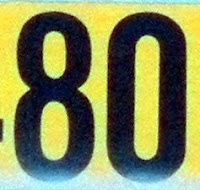Around the World in Eighty Days is a novel by Jules Verne.

## Monday, March 7, 2011

### 218

218 = 2 x 109.

218 has a representation as a sum of two squares: 218 = 72 + 132.

218 is the number of simple directed graphs of four nodes.

218 is 1333 in base 5, 1002 in base 6, 262 in base 9, and 118 in base 14.218 is the number of votes required in the United States House of Representatives to achieve a majority.

## Friday, March 4, 2011

### 166

166 = 2 x 83.

166 is the smallest number such that the number and its reversal are both congruent to 1 mod p for the same set of distinct odd primes: 166 = 661 = 1 mod (3, 5, 11).

166 is the smallest Smith number that is prime when reversed (661), turned upside down (991), and reversed-then-turned-upside-down (199). You get the next case by inserting a zero between the digits: 10606.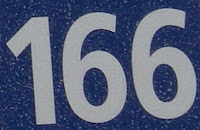U-166 was the only German submarine lost in the Gulf of Mexico.

## Thursday, March 3, 2011

### 772

772 = 22 x 193.

772 has a representation as a sum of two squares: 772 = 142 + 242.

772 is the sum of six distinct positive pentatope numbers.

772 is the sum of six positive cubes in exactly three ways.Telephone area code 772 serves that state of Florida.

## Wednesday, March 2, 2011

### 576

576 = 26 x 32.

576 has a unique representation as a sum of three squares: 576 = 82 + 162 + 162.

576 is the number of 4 x 4 Latin squares.

576 is the smallest square such that it remains a square when its leading digit is increased by 1: 676 = 262.

576 = (2 + 3 + 5 + 7 + 11)2 - (22 + 32 + 52 + 72 + 112).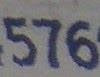New Balance produces a 576 line of fitness walking shoes.

## Tuesday, March 1, 2011

### 654

654 = 2 x 3 x 109.

654 has a square that is the sum of a cube and fifth power.

The sum 327 + 654 = 981 is one of only four cases in which the equality n + 2n = 3n uses each of the nine digits 1 to 9 once (n = 192, 219, 273, and 327).

654 is 545 in base 11.

654 is a Smith number. The sum of its digits is equal to the sum of the digits of its prime factors: 6 + 5 + 4 = 2 + 3 + 1 + 0 + 9 = 15.The Auburn Company produced the Auburn 654 automobile in 1936, the year the company went out of business.